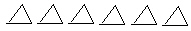Name:    2nd Grade Math Basic Test 2

Multiple Choice
Identify the choice that best completes the statement or answers the question.

1.

26
+5
 a. 21 d. 32 b. 11 e. 31 c. 20

2.

99
-56
 a. 34 d. 43 b. 155 e. 42 c. 53

3.

If Susan reads 3 more books, how many books will she read in all?
 a. 3 d. 6 b. 9 e. 8 c. 12

4.

834
-22
 a. 816 d. 756 b. 856 e. 812 c. 712

5.

Computation – Decimals – RIT 171 – 180
6.24
+2.53
 a. 877 d. 87.7 b. 8.78 e. 8.87 c. 8.77

6.

Computation – Fractions – RIT 171 – 180
3/5 + 1/5 =
 a. 3/5 d. 4/5 b. 3/25 e. 2/5 c. 4/10

7.

Computation – Whole Numbers – RIT 171 – 180
Juan had 24 crayons. He lost 10 of them.
How many crayons does Juan have now?
 a. 34 d. 18 b. 23 e. 10 c. 14

8.

36
+8
 a. 44 d. 45 b. 32 e. 34 c. 28

9.

321
+49
 a. 360 d. 328 b. 368 e. 370 c. 378

10.

78
-39
 a. 41 d. 27 b. 39 e. 47 c. 31

11.Choose the number sentence below that matches the picture.
 a. 4 X 6 d. 24 + 4 b. 6 + 4 e. 24 – 6 c. 4 X 24

12.

7,456
+4,872
 a. 12,428 d. 141,418 b. 12,328 e. 12,412 c. 12,318

13.

23
x 3
 a. 56 d. 59 b. 66 e. 68 c. 69

14.

46
x 2
 a. 68 d. 92 b. 102 e. 101 c. 82

15.

7 + 2 = 2 + ____
 a. 5 d. 11 b. 9 e. 7 c. 2